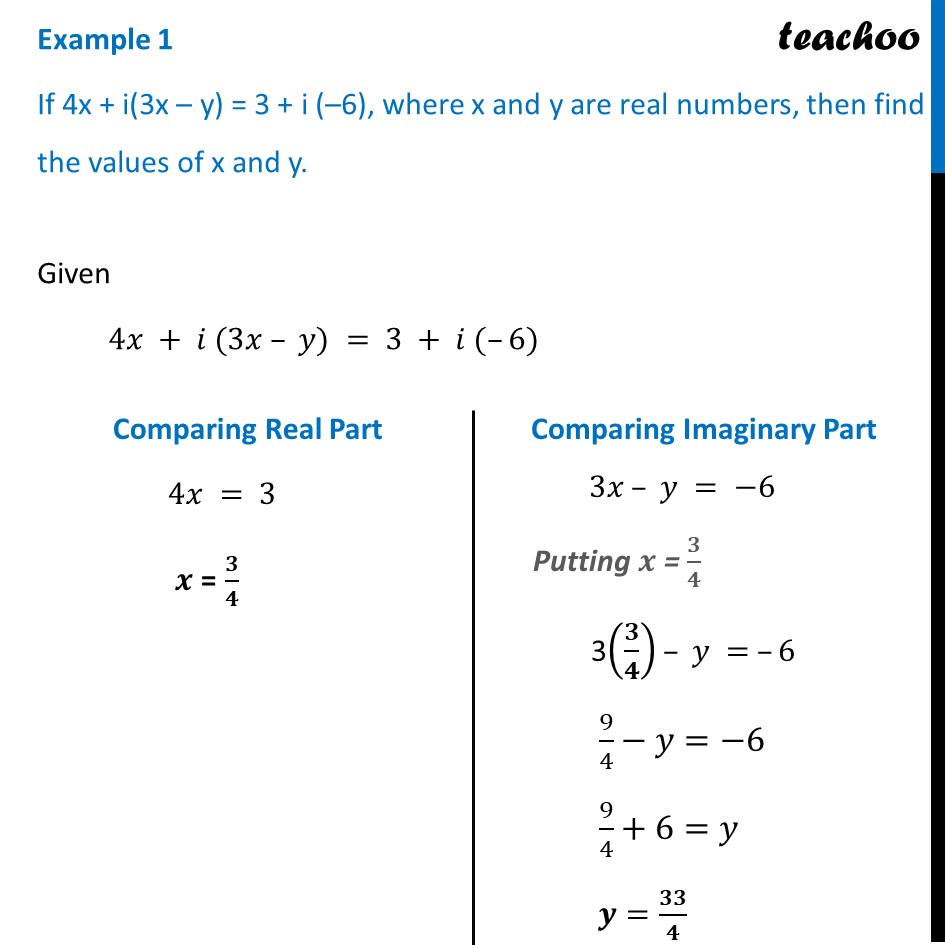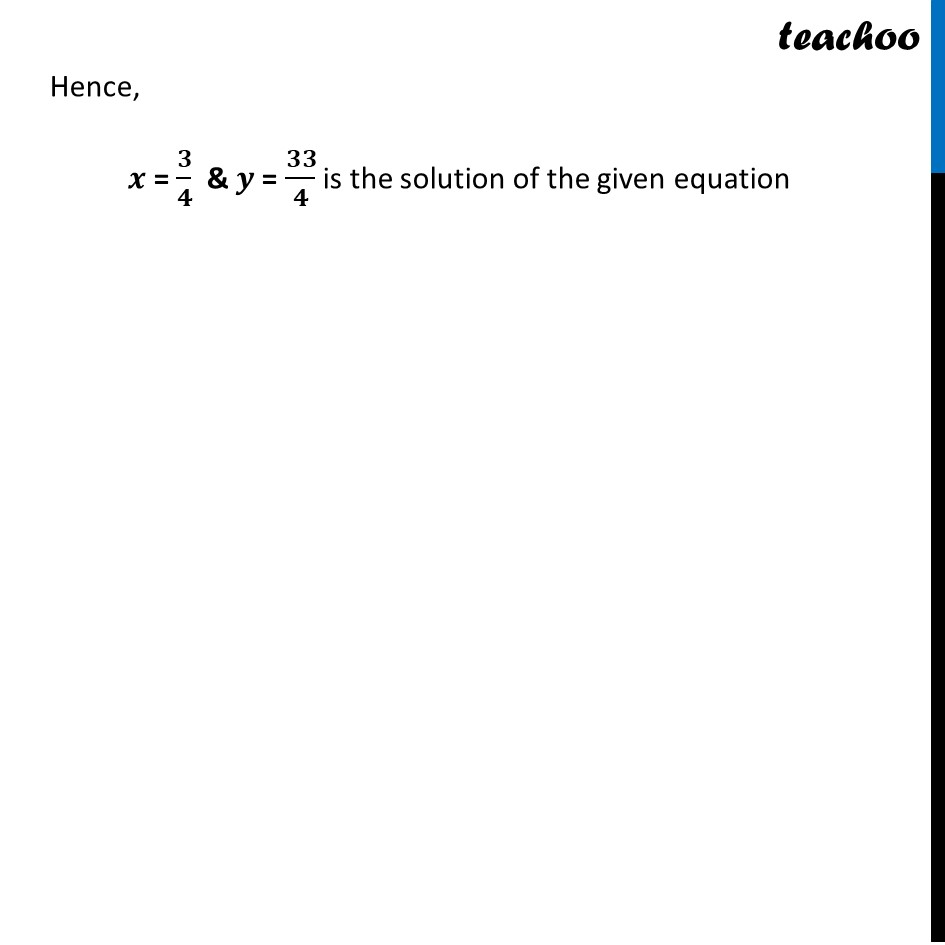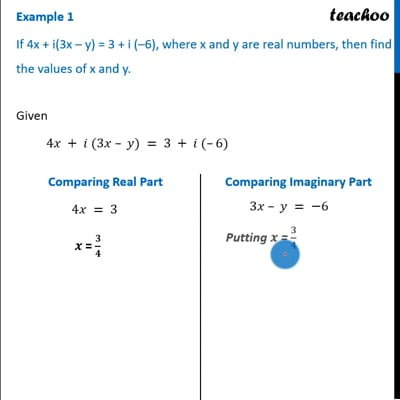Examples

Chapter 5 Class 11 Complex Numbers
Serial order wiseThis video is only available for Teachoo black users

Get live Maths 1-on-1 Classs - Class 6 to 12

### Transcript

Example 1 If 4x + i(3x – y) = 3 + i (–6), where x and y are real numbers, then find the values of x and y. Given 4𝑥 + 𝑖 (3𝑥 – 𝑦) = 3 + 𝑖 (–6) Comparing Real Part 4𝑥 = 3 𝒙 = 𝟑/𝟒 Comparing Imaginary Part 3𝑥 – 𝑦 = −6 Putting 𝒙 = 𝟑/𝟒 3(𝟑/𝟒) – 𝑦 = –6 9/4−𝑦=−6 9/4+6=𝑦 𝒚=𝟑𝟑/𝟒 Hence, 𝒙 = 𝟑/𝟒 & 𝒚 = 𝟑𝟑/𝟒 is the solution of the given equation